# Maharashtra Board 12th Maths Solutions Chapter 7 Linear Programming Ex 7.3

Balbharti 12th Maharashtra State Board Maths Solutions Book Pdf Chapter 7 Linear Programming Ex 7.3 Questions and Answers.

## Maharashtra State Board 12th Maths Solutions Chapter 7 Linear Programming Ex 7.3

Question 1.
A manufacturing firm produces two types of gadgets A and B, which are first processed in the foundry and then sent to a machine shop for finishing. The number of man-hours of labour required in each shop for production of A and B per unit and the number of man-hours available for the firm is as follows: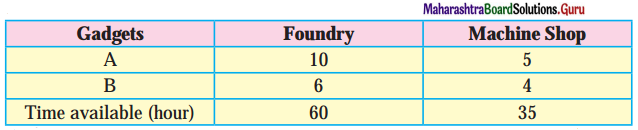Profit on the sale of A is ₹ 30 and B is ₹ 20 per units. Formulate the L.P.P. to have maximum profit.
Solution:
Let the number of gadgets A produced by the firm be x and the number of gadgets B produced by the firm be y.
The profit on the sale of A is ₹ 30 per unit and on the sale of B is ₹ 20 per unit.
∴ total profit is z = 30x + 20y.
This is a linear function which is to be maximized. Hence it is the objective function.
The constraints are as per the following table :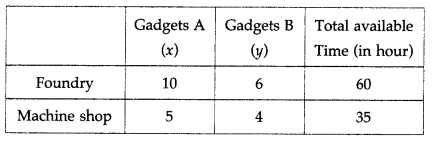From the table total man hours of labour required for x units of gadget A and y units of gadget B in foundry is (10x + 6y) hours and total man hours of labour required in machine shop is (5x + 4y) hours.
Since, maximum time avilable in foundry and machine shops are 60 hours and 35 hours respectively.
Therefore, the constraints are 10x + 6y ≤ 60, 5x + 4y ≤ 35. Since, x and y cannot be negative, we have x ≥ 0, y ≥ 0. Hence, the given LPP can be formulated as :
Maximize z = 30x + 20y, subject to 10x + 6y ≤ 60, 5x + 4y ≤ 35, x ≥ 0, y ≥ 0.

Question 2.
In a cattle breading firm, it is prescribed that the food ration for one animal must contain 14, 22 and 1 units of nutrients A, B and C respectively. Two different kinds of fodder are available. Each unit of these two contains the following amounts of these three nutrients :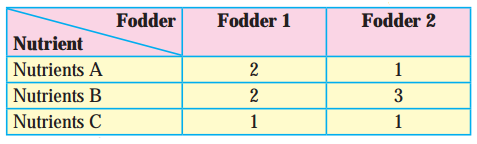The cost of fodder 1 is ₹3 per unit and that of fodder ₹ 2, Formulate the L.P.P. to minimize the cost.
Solution:
Let x units of fodder 1 and y units of fodder 2 be prescribed.
The cost of fodder 1 is ₹ 3 per unit and cost of fodder 2 is ₹ 2 per unit.
∴ total cost is z = 3x + 2y
This is the linear function which is to be minimized. Hence it is the objective function. The constraints are as per the following table :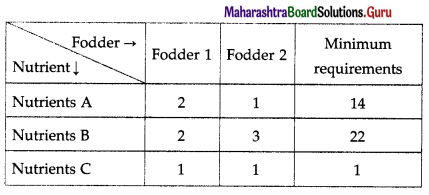From table fodder contains (2x + y) units of nutrients A, (2x + 3y) units of nutrients B and (x + y) units of nutrients C. The minimum requirements of these nutrients are 14 units, 22 units and 1 unit respectively.
Therefore, the constraints are
2x + y ≥ 14, 2x + 3y ≥ 22, x + y ≥ 1
Since, number of units (i.e. x and y) cannot be negative, we have, x ≥ 0, y ≥ 0.
Hence, the given LPP can be formulated as
Minimize z = 3x + 2y, subject to
2x + y ≥ 14, 2x + 3y ≥ 22, x + y ≥ 1, x ≥ 0, y ≥ 0.Question 3.
A company manufactures two types of chemicals A and B. Each chemical requires two types of raw material P and Q. The table below shows number of units of P and Q required to manufacture one unit of A and one unit of B and the total availability of P and Q.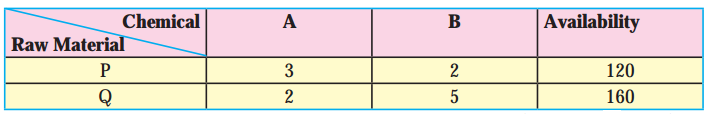The company gets profits of ₹350 and ₹400 by selling one unit of A and one unit of B respectively. (Assume that the entire production of A and B can be sold). How many units of the chemicals A and B should be manufactured so that the company get maximum profit? Formulate the problem as L.P.P. to maximize the profit.
Solution:
Let the company manufactures x units of chemical A and y units of chemical B. Then the total profit f to the company is p = ₹ (350x + 400y).
This is a linear function which is to be maximized.
Hence, it is the objective function.
The constraints are as per the following table: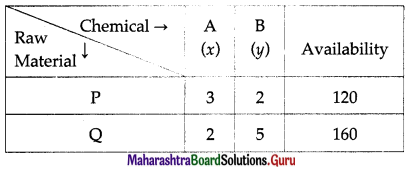The raw material P required for x units of chemical A and y units of chemical B is 3x + 2y. Since, the maximum availability of P is 120, we have the first constraint as 3x + 2y ≤ 120.
Similarly, considering the raw material Q, we have : 2x + 5y ≤ 160.
Since, x and y cannot be negative, we have, x ≥ 0, y ≥ 0.
Hence, the given LPP can be formulated as :
Maximize p = 350x + 400y, subject to
3x + 2y ≤ 120, 2x + 5y ≤ 160, x ≥ 0, y ≥ 0.

Question 4.
A printing company prints two types of magazines A and B. The company earns ₹ 10 and ₹ 15 on magazines A and B per copy. These are processed on three machines I, II, III. Magazine A requires 2 hours on Machine I, 5 hours on Machine II and 2 hours on Machine III. Magazine B requires 3 hours on Machine I, 2 hours on Machine II and 6 hours on Machine III. Machines I, II, III are available for 36, 50, 60 hours per week respectively. Formulate the L.P.P. to determine weekly production of A and B, so that the total profit is maximum.
Solution:
Let the company prints x magazine of type A and y magazine of type B.
Profit on sale of magazine A is ₹ 10 per copy and magazine B is ₹ 15 per copy.
Therefore, the total earning z of the company is
z = ₹ (10x + 15y).
This is a linear function which is to be maximized.
Hence, it is the objective function.
The constraints are as per the following table: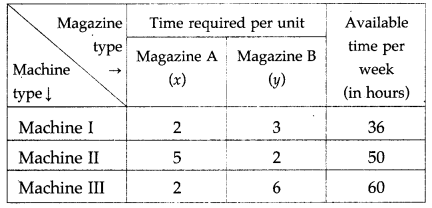From the table, the total time required for Machine I is (2x + 3y) hours, for Machine II is (5x + 2y) hours and for Machine III is (2x + 6y) hours. The machines I, II, III are available for 36,50 and 60 hours per week. Therefore, the constraints are 2x + 3y ≤ 36, 5x + 2y ≤ 50, 2x + 6y ≤ 60.
Since x and y cannot be negative. We have, x ≥ 0, y ≥ 0. Hence, the given LPP can be formulated as :
Maximize z = 10x + 15y, subject to
2x + 3y ≤ 36, 5x + 2y ≤ 50, 2x + 6y ≤ 60, x ≥ 0, y ≥ 0.Question 5.
A manufacture produces bulbs and tubes. Each of these must be processed through two machines M1 and M2. A package of bulbs require 1 hour of work on Machine M1 and 3 hours of work on M2. A package of tubes require 2 hours on Machine M1 and 4 hours on Machine M2. He earns a profit of ₹ 13.5 per package of bulbs and ₹ 55 per package of tubes. Formulate the LLP to maximize the profit, if he operates the machine M1, for atmost 10 hours a day and machine M2 for atmost 12 hours a day.
Solution:
Let the number of packages of bulbs produced by manufacturer be x and packages of tubes be y. The manufacturer earns a profit of ₹ 13.5 per package of bulbs and ₹ 55 per package of tubes.
Therefore, his total profit is p = ₹ (13.5x + 55y)
This is a linear function which is to be maximized.
Hence, it is the objective function.
The constraints are as per the following table :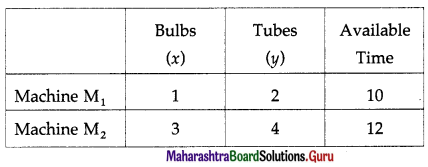From the table, the total time required for Machine M1 is (x + 2y) hours and for Machine M2 is (3x + 4y) hours.
Given Machine M1 and M2 are available for atmost 10 hours and 12 hours a day respectively.
Therefore, the constraints are x + 2y ≤ 10, 3x + 4y ≤ 12. Since, x and y cannot be negative, we have, x ≥ 0, y ≥ 0. Hence, the given LPP can be formulated as :
Maximize p = 13.5x + 55y, subject to x + 2y ≤ 10, 3x + 4y ≤ 12, x ≥ 0, y ≥ 0.

Question 6.
A company manufactures two types of fertilizers F1 and F2. Each type of fertilizer requires
two raw materials A and B. The number of units of A and B required to manufacture one unit of fertilizer F1 and F2 and availability of the raw materials A and B per day are given in the
table below :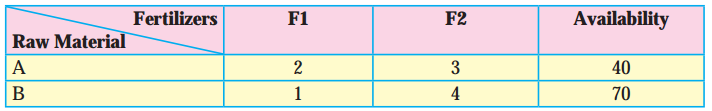By selling one unit of F1 and one unit of F2, company gets a profit of ₹ 500 and ₹ 750
respectively. Formulate the problem as L.P.P. to maximize the profit.
Solution:
Let the company manufactures x units of fertilizers F1 and y units of fertilizers F1. Then the total profit to the company is
z = ₹(500x + 750y).
This is a linear function that is to be maximized. Hence, it is an objective function.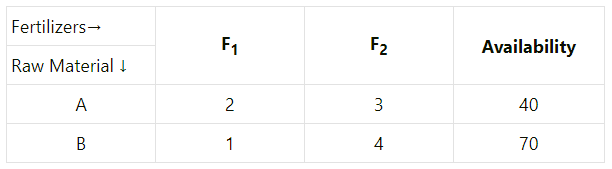The raw material A required for x units of Fertilizers F1 and y units of Fertilizers F2 is 2x + Since the maximum availability of A is 40, we have the first constraint as 2x + 3y ≤ 40.
Similarly, considering the raw material B, we have x + 4y ≤ 70.
Since, x and y cannot be negative, we have, x ≥ 0, y ≥ 0.
Hence, the given LPP can be formulated as:
Maximize z = 500x + 750y, subject to
2x + 3y ≤ 40, x + 4y ≤ 70, x ≥ 0, y ≥ 0.Question 7.
A doctor has prescribed two different units of foods A and B to form a weekly diet for a sick person. The minimum requirements of fats, carbohydrates and proteins are 18, 28, 14 units respectively. One unit of food A has 4 units of fats. 14 units of carbohydrates and 8 units of protein. One unit of food B has 6 units of fat, 12 units of carbohydrates and 8 units of protein. The price of food A is ₹ 4.5 per unit and that of food B is ₹ 3.5 per unit. Form the L.P.P. so that the sick person’s diet meets the requirements at a minimum cost.
Solution:
Let the diet of sick person include x units of food A and y units of food B.
Then x ≥ 0, y ≥ 0.
The prices of food A and B are ₹ 4.5 and ₹ 3.5 per unit respectively.
Therefore, the total cost is z = ₹ (4.5x + 3.5y)
This is the linear function which is to be minimized.
Hence, it is objective function.
The constraints are as per the following table :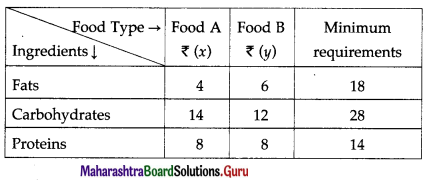From the table, the sick person’s diet will include (4x + 6y) units of fats, (14x + 12y) units of carbohydrates and (8x + 8y) units of proteins. The minimum requirements of these ingredients are 18 units, 28 units and 14 units respectively.
Therefore, the constraints are
4x + 6y ≥ 18, 14x + 12y ≥ 28, 8x + 8y ≥ 14.
Hence, the given LPP can be formulated as
Minimize z = 4.5x + 3.5y, subject to
4x + 6y ≥ 18, 14x + 12y ≥ 28, 8x + 8y ≥ 14, x ≥ 0, y ≥ 0.

Question 8.
If John drives a car at a speed of 60 kms/hour he has to spend ₹ 5 per km on petrol. If he drives at a faster speed of 90 kms/hour, the cost of petrol increases to ₹ 8 per km. He has ₹ 600 to spend on petrol and wishes to travel the maximum distance within an hour. Formulate the above problem as L.P.P.
Solution:
Let John travel xl km at a speed of 60 km/ hour and x1 km at a speed of 90 km/hour.
Therefore, time required to travel a distance of x1 km is $$\frac{x_{1}}{60}$$ hours and the time required to travel a distance of
x2 km is $$\frac{x_{2}}{90}$$ hours.
∴ total time required to travel is $$\left(\frac{x_{1}}{60}+\frac{x_{2}}{90}\right)$$ hours.
Since he wishes to travel the maximum distance within an hour,
$$\frac{x_{1}}{60}+\frac{x_{2}}{90}$$ ≤ 1
He has to spend ₹ 5 per km on petrol at a speed of 60 km/hour and ₹ 8 per km at a speed of 90 km/hour.
∴ the total cost of travelling is ₹ (5x1 + 8x2)
Since he has ₹ 600 to spend on petrol,
5x1 + 8x2 ≤ 600
Since distance is never negative, x1 ≥ 0, x2 ≥ 0.
Total distance travelled by John is z. = (x1 + x2) km.
This is the linear function which is to be maximized.
Hence, it is objective function.
Hence, the given LPP can be formulated as :
Maximize z = x1 + x2, subject to
$$\frac{x_{1}}{60}+\frac{x_{2}}{90}$$ ≤ 1, 5x1 + 8x2 ≤ 600, x1 ≥ 0, x2 ≥ 0.Question 9.
The company makes concrete bricks made up of cement and sand. The weight of a concrete brick has to be least 5 kg. Cement costs ₹ 20 per kg. and sand costs of ₹ 6 per kg. strength consideration dictate that a concrete brick should contain minimum 4 kg. of cement and not more than 2 kg. of sand. Form the L.P.P. for the cost to be minimum.
Solution:
Let the company use x1 kg of cement and x2 kg of sand to make concrete bricks.
Cement costs ₹ 20 per kg and sand costs ₹ 6 per kg.
∴ the total cost c = ₹ (20x1 + 6x2)
This is a linear function which is to be minimized.
Hence, it is the objective function.
Total weight of brick = (x1 + x2) kg
Since the weight of concrete brick has to be at least 5 kg,
∴ x1 + x2 ≥ 5.
Since concrete brick should contain minimum 4 kg of cement and not more than 2 kg of sand,
x1 ≥ 4 and 0 ≤ x2 ≤ 2
Hence, the given LPP can be formulated as :
Minimize c = 20x1 + 6x2, subject to
x1 + x2 ≥ 5, x1 ≥ 4, 0 ≤ x2 ≤ 2.